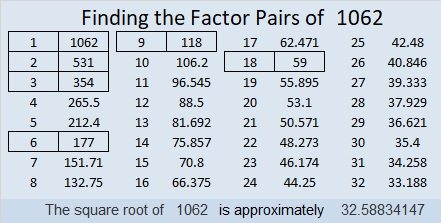# 1062 Complicated Logic

The logic used to solve this particular level 6 puzzle is complicated, but answering the following questions in the order given will help you to see and understand that logic:

1. Two of the numbers from 1 to 10 have only one clue each in this puzzle. What are those two numbers? The product of those two numbers is the missing clue that you will use later in the puzzle.
2. Which two clues MUST use both 1’s?
3. Which four clues must use all the 3’s and 6’s?
4. Can both 30’s be 3 × 10 or be 5 × 6?
5. Can both 40’s be 4 × 10 or be 5 × 8?
6. What MUST be the factors of 24 in this puzzle?
7. What clues must use both 4’s? What clues must use both 8s?
8. Is 1 or 2 the common factor for clues 8 and 2 that will make the puzzle work?
9. Is 5 or 10 the common factor for clues 30 and 40 near the bottom of the puzzle?

Once you know the answers to those questions and the two sets of common factors, you can very quickly complete the puzzle.Print the puzzles or type the solution in this excel file: 10-factors-1054-1062

Here’s some information about the number 1062:

• 1062 is a composite number.
• Prime factorization: 1062 = 2 × 3 × 3 × 59, which can be written 1062 = 2 × 3² × 59
• The exponents in the prime factorization are 1, 2, and 1. Adding one to each and multiplying we get (1 + 1)(2 + 1)(1 + 1) = 2 × 3 × 2 = 12. Therefore 1062 has exactly 12 factors.
• Factors of 1062: 1, 2, 3, 6, 9, 18, 59, 118, 177, 354, 531, 1062
• Factor pairs: 1062 = 1 × 1062, 2 × 531, 3 × 354, 6 × 177, 9 × 118, or 18 × 59,
• Taking the factor pair with the largest square number factor, we get √1062 = (√9)(√118) = 3√118 ≈ 32.588341062 looks interesting when it is written in a couple of different bases:
It’s 2D2 in BASE 20 (D is 13 base 10) because 2(20²) + 13(20) + 2(1) = 1062
and 246 in BASE 22 because 2(22²) + 4(22) + 6(1) = 1062

Advertisements

This site uses Akismet to reduce spam. Learn how your comment data is processed.